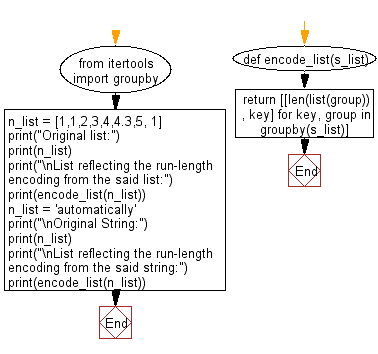﻿ Python: Create a list reflecting the run-length encoding from a list - w3resource# Python: Create a list reflecting the run-length encoding from a list

## Python List: Exercise - 75 with Solution

Run-length encoding (RLE) is a form of lossless data compression in which runs of data (sequences in which the same data value occurs in many consecutive data elements) are stored as a single data value and count, rather than as the original run.

Write a Python program to create a list reflecting the run-length encoding from a given list of integers or a given list of characters.

Sample Solution:

Python Code:

``````from itertools import groupby
def encode_list(s_list):
return [[len(list(group)), key] for key, group in groupby(s_list)]
n_list = [1,1,2,3,4,4.3,5, 1]
print("Original list:")
print(n_list)
print("\nList reflecting the run-length encoding from the said list:")
print(encode_list(n_list))
n_list = 'automatically'
print("\nOriginal String:")
print(n_list)
print("\nList reflecting the run-length encoding from the said string:")
print(encode_list(n_list))
```
```

Sample Output:

```Original list:
[1, 1, 2, 3, 4, 4.3, 5, 1]

List reflecting the run-length encoding from the said list:
[[2, 1], [1, 2], [1, 3], [1, 4], [1, 4.3], [1, 5], [1, 1]]

Original String:
automatically

List reflecting the run-length encoding from the said string:
[[1, 'a'], [1, 'u'], [1, 't'], [1, 'o'], [1, 'm'], [1, 'a'], [1, 't'], [1, 'i'], [1, 'c'], [1, 'a'], [2, 'l'], [1, 'y']]
```

Flowchart:## Visualize Python code execution:

The following tool visualize what the computer is doing step-by-step as it executes the said program:

Python Code Editor:

Have another way to solve this solution? Contribute your code (and comments) through Disqus.

What is the difficulty level of this exercise?

Test your Python skills with w3resource's quiz

﻿

## Python: Tips of the Day

Floor Division:

When we speak of division we normally mean (/) float division operator, this will give a precise result in float format with decimals.

For a rounded integer result there is (//) floor division operator in Python. Floor division will only give integer results that are round numbers.

```print(1000 // 300)
print(1000 / 300)```

Output:

```3
3.3333333333333335```